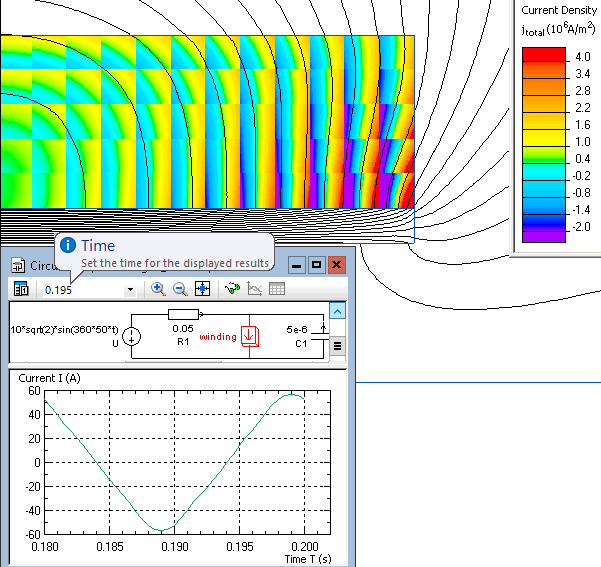Main >> Applications >> Sample problems

# Coil with ferromagnetic core

ferromagnetic core solenoid transient current, coil winding non-sinusoidal current

This is an example of the coil with ferromagnetic core simulation, performed with QuickField software.

Problem Type
Axisymmetric problem of transient magnetics.

Geometry

Given
Conductivity of the steel core g = 10 MS/m;
Conductivity of the winding (copper) g = 56 MS/m;
Number of turns w = 120;
Applied voltage value (amplitude) U = 10√2 V;
Frequency f = 50 Hz.
Magnetic permeability of the steel core μ - nonlinear:

Determine the electric current within the coil winding.

Solution
Due to the model symmetry only the right half of the coil is presented in the model (shown by upper half of its cross-section). Therefore the circuit elements' values are defined twice less than in the real object.
The current wave period is T = 1 / f = 0.02 sec. We choose the time step of 0.0005 second that guarantees a smooth plot. The finishing time 0.2 sec includes 10 periods and is long enough to fade out initial transient currents. To reduce the results file size only the last period is stored (starting from moment 0.18 sec).

Results
The fundamental harmonic approximation of the current in the winding is: I(t) = 51.5 · sin(t·2π/T + 108°)• Video: Coil with ferromagnetic core
•